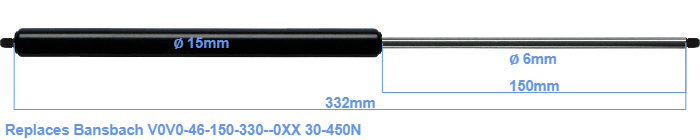# Replacement for Bansbach V0V0-46-150-330–0XX 30-450N

\$27.12

Replacement gas spring for the Bansbach V0V0-46-150-330--0XX 30-450 Newton. Thread: M5. Brand: HAHN, a Stabilus company.
 Force Choose an option30 Newton40 Newton50 Newton60 Newton80 Newton100 Newton120 Newton140 Newton150 Newton160 Newton180 Newton200 Newton220 Newton240 Newton250 Newton260 Newton280 Newton300 Newton320 Newton340 Newton350 Newton360 Newton380 Newton400 Newton420 Newton440 Newton450 NewtonClearThis gas spring has a cylinder diameter of 15 mm. The diameter of the rod totals 6 mm. The rod (the chrome part) is 150 mm long. The total length of this replacement is exactly 332 mm. Note: this is the total length between the rotation points of the two mounting parts. The length from thread to thread (exlcuding the mounting parts) is 332 millimeter. This replacement gas spring (V0V0-46-150-330--0XX) has a force of 30-450 Newton (N). This is not an official Bansbach gas spring, but a so called replacement. This is a HAHN gas spring. The force (30-450 N) and dimensions are identical to the original.